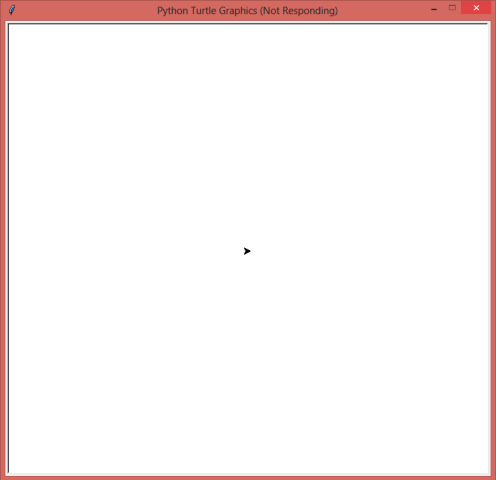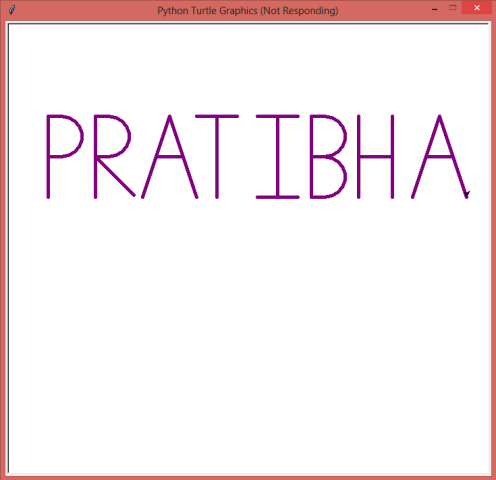top of page
Search

# Turtle Graphics: Make the turtle write your name and much more.

Turtle graphics is a built-in python module that provides a canvas and a turtle (cursor) to let you show your creativity. The turtle moves around the canvas and draws as directed.The canvas can be thought of as a graph having the origin(0,0) at its very centre. The centre is called home. That way we can assume that the canvas is divided into four quadrants.The turtle is a cursor that moves over the canvas following the instructions from the user. Initially it rests at home. When given a command turtle.forward(20), it moves 20 units in the direction in which it is pointing while drawing a line. When given a command turtle.left(90), it will rotate 90 degrees in the left direction while still being in-place. By using many other commands as mentioned above we can design many shapes and images easily.

Check out the documentation for Turtle Graphics here: https://docs.python.org/3/library/turtle.html#turtle.goto

Below are some of the commands that we will be using in the program code:

• turtle.reset(): It deletes the drawings of the turtle and sends the turtle back to home and sets everything to default.

• turtle.write(arg, move=False, align="left", font=("Arial", 8, "normal")): It writes the string passed in arg on the screen. The text can be formatted with align (“left”, “centre” or right”) and font(style,size,(“normal”,”bold”,”italic”)). If move is true, the pen is moved to the bottom-right corner of the text. By default, move is False.

• turtle.pencolor(): It sets the pencolor. It allows four types of arguments.

• turtle.pensize(width): It sets the thickness of the line drawn.

• turtle.penup() or turtle.pu(): It pulls the pen up and doesn’t draw while moving.

• turtle.pendown() or turtle.pd(): It pulls the pen down and draws while moving.

• turtle.goto(x,y) : It moves turtle to an absolute position specified by values of x and y coordinates without changing the turtle’s orientation.

• turtle.forward(distance) or turtle.fd(distance): It moves the turtle forward in the direction which it is pointing to by the specified distance.

• turtle.backward(distance) or turtle.bk(distance): It moves the turtle backward (opposite to the direction in which it is pointing) by the specified distance without changing its orientation

• turtle.right(angle) or turtle.rt(angle): It rotates the turtle to its right by the specified angle.

• turtle.left(angle) or turtle.rt(angle): It rotates the turtle to its left by the specified angle.

• turtle.circle(radius, extent=None, steps=None): It draws a circle with a given radius and extent. If extent is not given, it draws the entire circle.

Now, using the above commands we will make the turtle write our name. It's like guiding a blind-folded person to reach his/her destination.

It is better to plan out beforehand on a sheet of paper keeping the coordinates in mind to get a result which looks uniform.

Moving ahead there are two ways to write a text using the turtle.

• The first method is to use turtle.write() function. It is the easier way.

• First we import the turtle library.

`	import turtle			`
• Then, we set the colour and style of the text. We use turtle.write() and pass the string containing name.

```        turtle.color('purple')
style = ('Courier', 90, 'normal')
turtle.write('PRATIBHA', font=style, align='center')
turtle.hideturtle()	   ```

This will print the name string on the turtle screen. The output is given below:To delete the drawing by the turtle use: turtle.reset()

• The second method requires a lot of planning and hence is a bit tedious, but all the more fun. We guide the turtle to draw.

• First we import the turtle library.

`	import turtle`
• Then we assign the turtle a new name, say 't'.

`        t = turtle.Turtle()`
• Then we set the size and color of the pen and move the turtle (without drawing i.e. wit t.penup() ) to a specific point from where we will start drawing.

```        t.reset()
t.pencolor('purple')
t.pensize(5)
t.penup()
t.goto(-300,200)```
• From here on, we will move the turtle forward, backward, right or left in such a way that we get the desired output. Below is an example to draw the letters 'P' and 'R', though various other codes can be used to produce the same result.

```        #p
t.pendown()
t.fd(20)
t.circle(-30, 180)
t.fd(20)
t.rt(90)
t.fd(60)
t.bk(60)
t.lt(180)
t.fd(60)

t.penup()
t.goto(-230,200)

#R
t.pendown()
t.lt(90)
t.fd(20)
t.circle(-30,180)
t.fd(20)
t.rt(90)
t.fd(60)
t.bk(60)
t.lt(180)
t.fd(60)
t.bk(60)
t.lt(45)
t.fd(80)
t.rt(45)

t.penup()
t.goto(-160,200)```
• This way we write all the other letters of the name.

The output for the full name is given below:We can draw many figures, whether easy or complex using the combination of above commands. Try your hand at it, it is fun.